### Home > CALC > Chapter 10 > Lesson 10.1.4 > Problem10-32

10-32.
1. Without your calculator, determine if the following integrals are finite or not. Homework Help ✎1.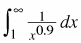2.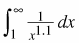3.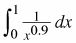4.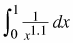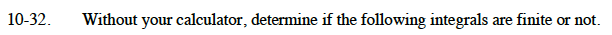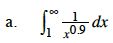$\lim_{a\to\infty}\int_1^a\frac{1}{x^{0.9}}dx=\lim_{a\to\infty}\int_{1}^{a}x^{-0.9}dx$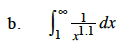See the step in part (a).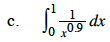$\lim_{c\to 0}\int_c^1\frac{1}{x^{0.9}}dx=\lim_{c\to 0}\int_{c}^{1}x^{-0.9}dx$See the step in part (c).